# Python | Arithmetic operations in excel file using openpyxl

Prerequisite: Reading & Writing to excel sheet using openpyxl
Openpyxl is a Python library using which one can perform multiple operations on excel files like reading, writing, arithmetic operations and plotting graphs. Let’s see how to perform different arithmetic operations using openpyxl.

• =SUM(cell1:cell2) : Adds all the numbers in a range of cells.

## Python3

 `# import openpyxl module` `import` `openpyxl`   `# Call a Workbook() function of openpyxl ` `# to create a new blank Workbook object` `wb ``=` `openpyxl.Workbook()`   `# Get workbook active sheet  ` `# from the active attribute.` `sheet ``=` `wb.active`   `# writing to the cell of an excel sheet` `sheet[``'A1'``] ``=` `200` `sheet[``'A2'``] ``=` `300` `sheet[``'A3'``] ``=` `400` `sheet[``'A4'``] ``=` `500` `sheet[``'A5'``] ``=` `600`   `# The value in cell A7 is set to a formula ` `# that sums the values in A1, A2, A3, A4, A5 .` `sheet[``'A7'``] ``=` `'= SUM(A1:A5)'`   `# save the file` `wb.save(``"sum.xlsx"``)`

• Output: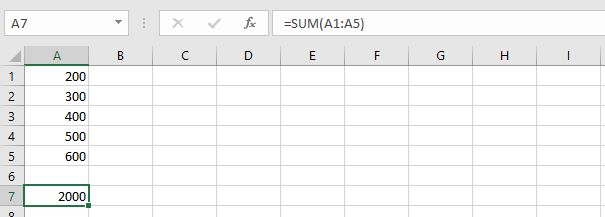• =PRODUCT(cell1:cell2) : Multiplies all the numbers in the range of cells.

## Python3

 `import` `openpyxl`   `wb ``=` `openpyxl.Workbook()` `sheet ``=` `wb.active`   `sheet[``'A1'``] ``=` `2` `sheet[``'A2'``] ``=` `3` `sheet[``'A3'``] ``=` `4` `sheet[``'A4'``] ``=` `5` `sheet[``'A5'``] ``=` `6`   `# The value in cell A7 is set to a formula ` `# that multiplies the values in A1, A2, A3, A4, A5 .` `sheet[``'A7'``] ``=` `'= PRODUCT(A1:A5)'`   `wb.save(``"product.xlsx"``)`

• Output: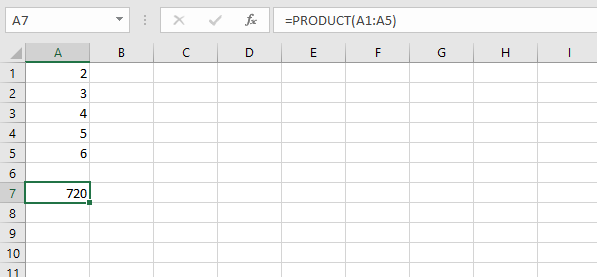• =AVERAGE(cell1:cell2) : It gives the average (arithmetical mean) of all the numbers which is present in the given cell range.

## Python3

 `import` `openpyxl`   `wb ``=` `openpyxl.Workbook()` `sheet ``=` `wb.active`   `sheet[``'A1'``] ``=` `200` `sheet[``'A2'``] ``=` `300` `sheet[``'A3'``] ``=` `400` `sheet[``'A4'``] ``=` `500` `sheet[``'A5'``] ``=` `600`   `# The value in cell A7 is set to a formula ` `# that return average of the values in A1, A2, A3, A4, A5 .` `sheet[``'A7'``] ``=` `'= AVERAGE(A1:A5)'`   `wb.save(``"average.xlsx"``)`

• Output: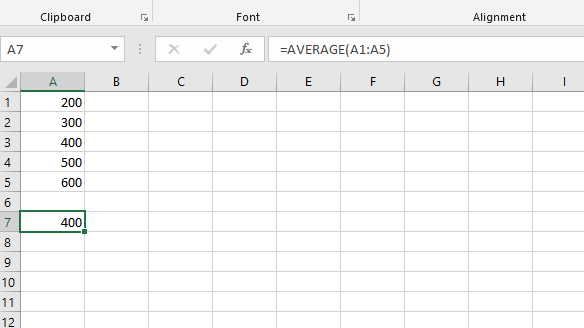• =QUOTIENT(num1, num2) : It returns the integer portion of a division.

## Python3

 `import` `openpyxl`   `wb ``=` `openpyxl.Workbook()` `sheet ``=` `wb.active`   `# The value in cell is set to a formula ` `# that gives quotient value .` `sheet[``'A1'``] ``=` `'= QUOTIENT(64, 8)'` `sheet[``'A2'``] ``=` `'= QUOTIENT(25, 4)'`   `wb.save(``"quotient.xlsx"``)`

• Output: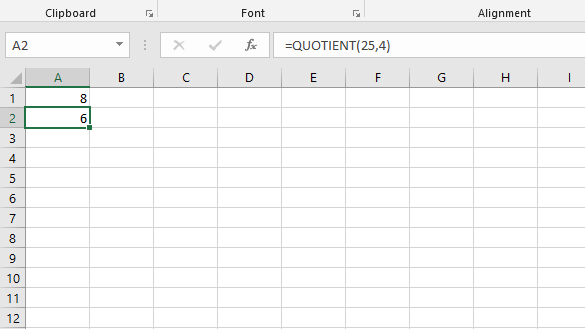• =MOD(num1, num2) : Returns the remainder after a number is divided by the divisor.

## Python3

 `import` `openpyxl`   `wb ``=` `openpyxl.Workbook()` `sheet ``=` `wb.active`   `# The value in cell is set to a formula ` `# that gives remainder or modulus value.` `sheet[``'A1'``] ``=` `'= MOD(64, 8)'` `sheet[``'A2'``] ``=` `'= MOD(25, 4)'`   `wb.save(``"modulus.xlsx"``)`

• Output: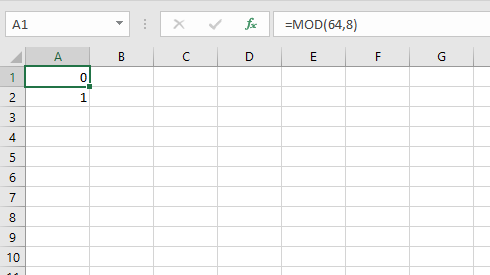• =COUNT(cell1:cell2) : It counts the number of cells in a range that contain the number.

## Python3

 `import` `openpyxl`   `wb ``=` `openpyxl.Workbook()` `sheet ``=` `wb.active`   `sheet[``'A1'``] ``=` `200` `sheet[``'A2'``] ``=` `300` `sheet[``'A3'``] ``=` `400` `sheet[``'A4'``] ``=` `500` `sheet[``'A5'``] ``=` `600`   `# The value in cell A7 is set to a formula ` `# that gives counting of number present in the cells.` `sheet[``'A7'``] ``=` `'= COUNT(A1:A6)'`   `wb.save(``"count.xlsx"``)`

• Output: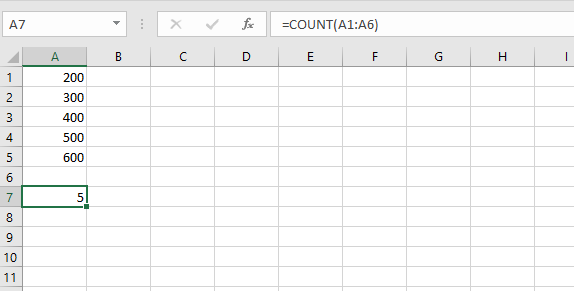Whether you're preparing for your first job interview or aiming to upskill in this ever-evolving tech landscape, GeeksforGeeks Courses are your key to success. We provide top-quality content at affordable prices, all geared towards accelerating your growth in a time-bound manner. Join the millions we've already empowered, and we're here to do the same for you. Don't miss out - check it out now!

Previous
Next